Скачать презентацию CPSC 601 Lecture Week 5 Hand Geometry

c9c400d54aaaac26e86556e03909ccdd.ppt

• Количество слайдов: 31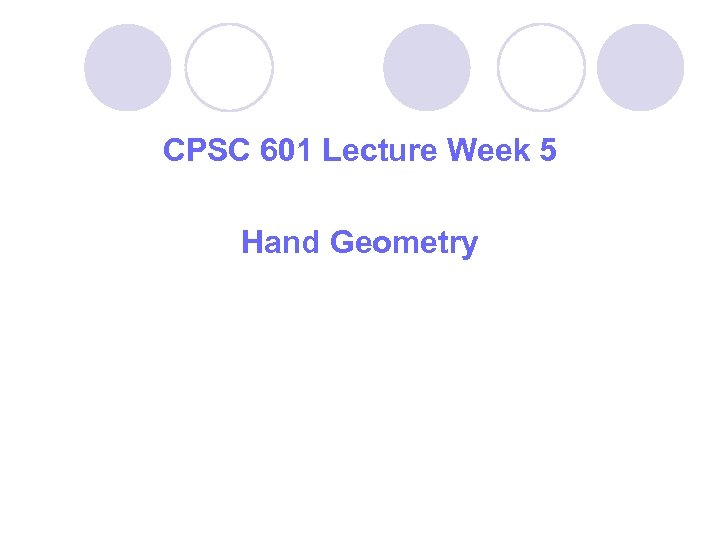CPSC 601 Lecture Week 5 Hand Geometry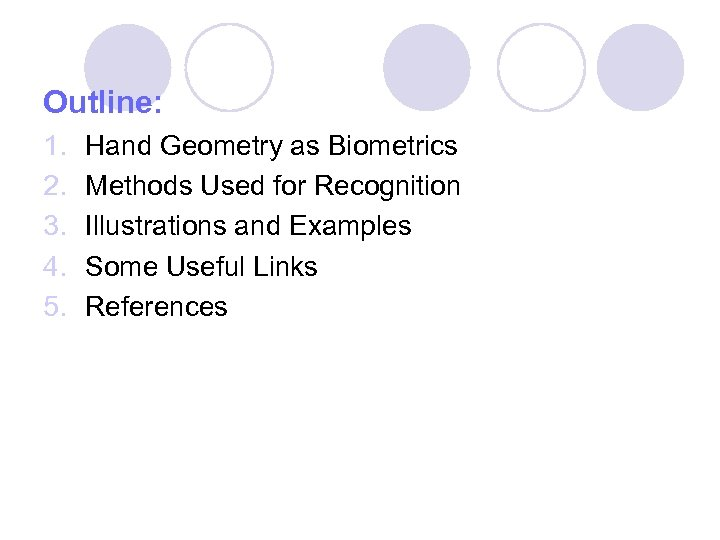Outline: 1. 2. 3. 4. 5. Hand Geometry as Biometrics Methods Used for Recognition Illustrations and Examples Some Useful Links References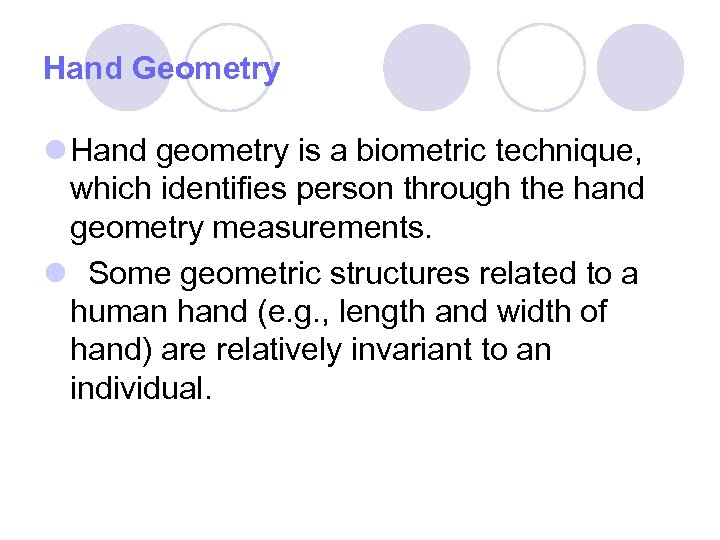Hand Geometry l Hand geometry is a biometric technique, which identifies person through the hand geometry measurements. l Some geometric structures related to a human hand (e. g. , length and width of hand) are relatively invariant to an individual.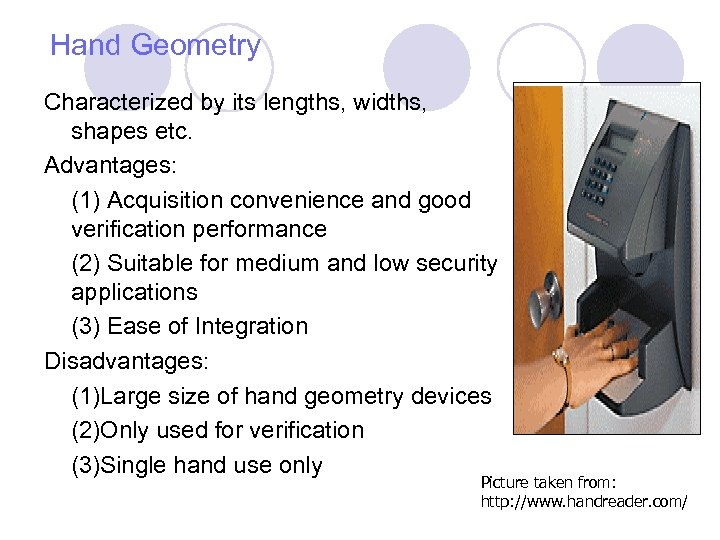Hand Geometry Characterized by its lengths, widths, shapes etc. Advantages: (1) Acquisition convenience and good verification performance (2) Suitable for medium and low security applications (3) Ease of Integration Disadvantages: (1)Large size of hand geometry devices (2)Only used for verification (3)Single hand use only Picture taken from: http: //www. handreader. com/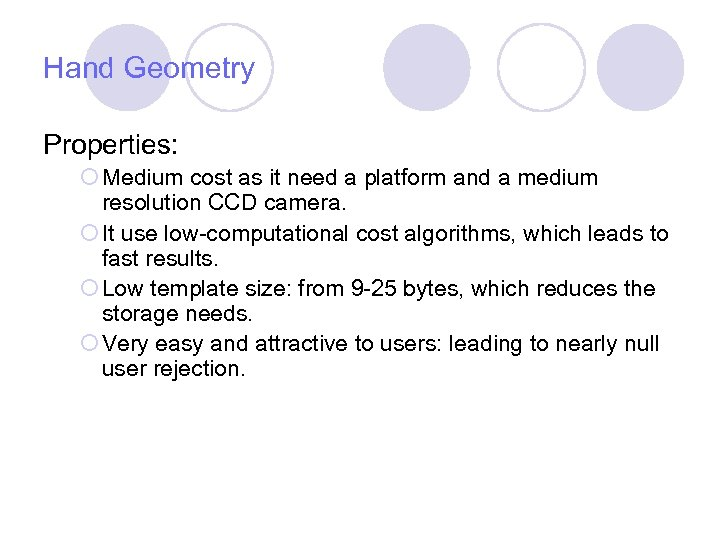Hand Geometry Properties: ¡ Medium cost as it need a platform and a medium resolution CCD camera. ¡ It use low-computational cost algorithms, which leads to fast results. ¡ Low template size: from 9 -25 bytes, which reduces the storage needs. ¡ Very easy and attractive to users: leading to nearly null user rejection.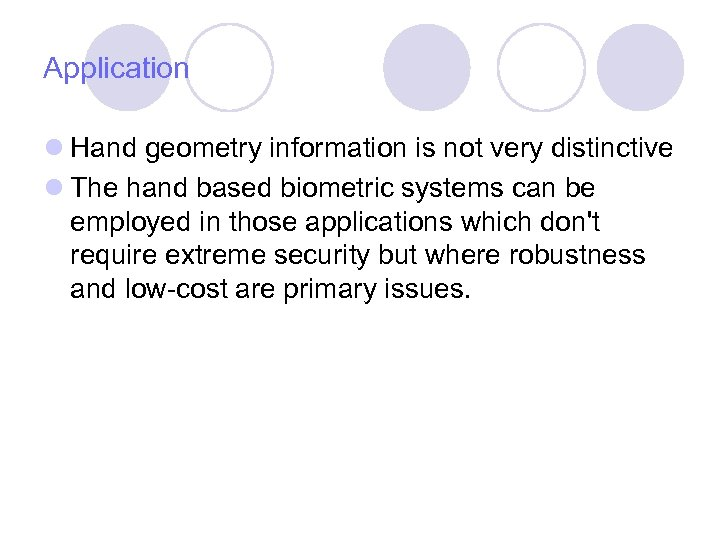Application l Hand geometry information is not very distinctive l The hand based biometric systems can be employed in those applications which don't require extreme security but where robustness and low-cost are primary issues.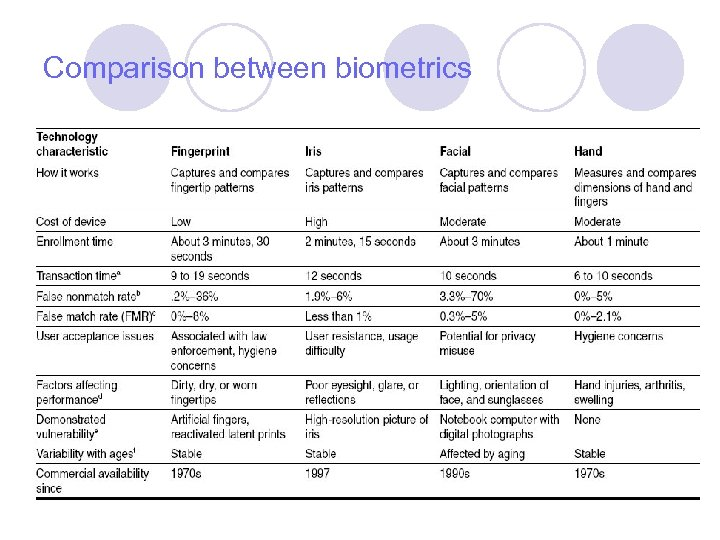Comparison between biometrics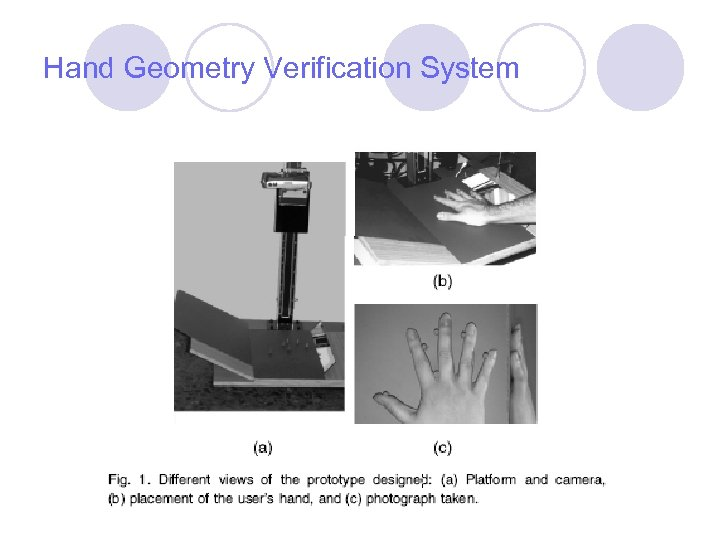Hand Geometry Verification System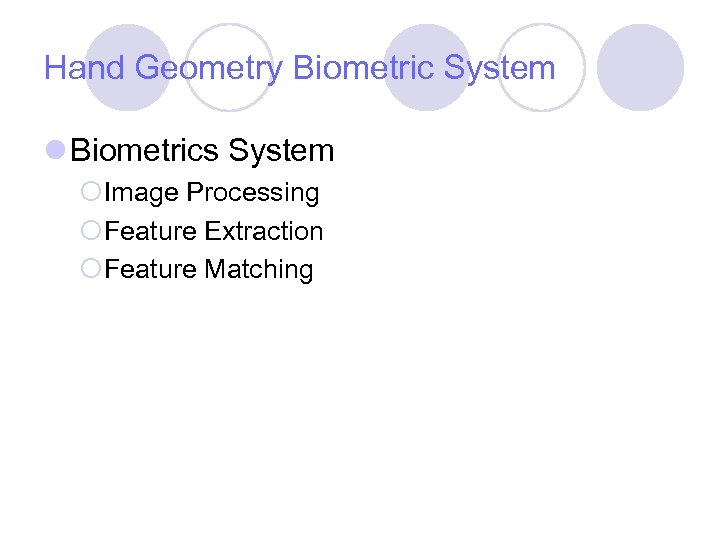Hand Geometry Biometric System l Biometrics System ¡Image Processing ¡Feature Extraction ¡Feature Matching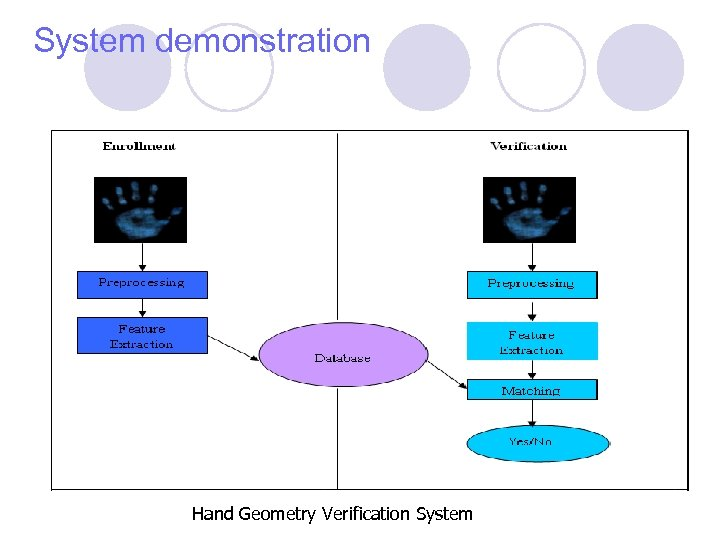System demonstration Hand Geometry Verification System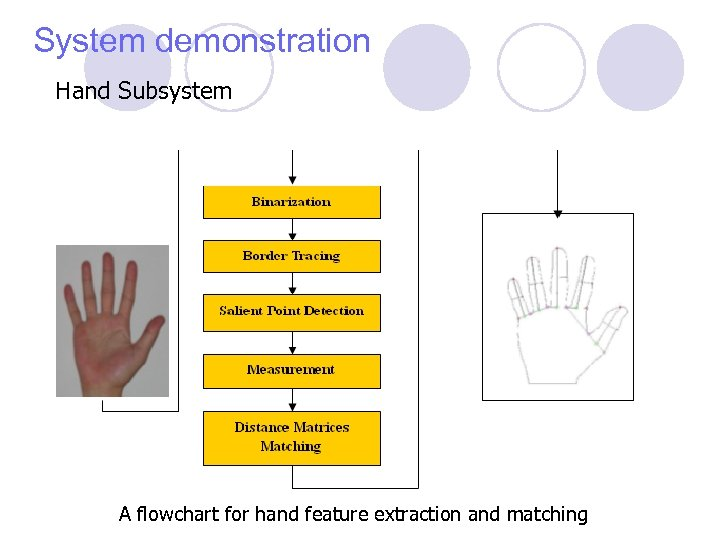System demonstration Hand Subsystem A flowchart for hand feature extraction and matching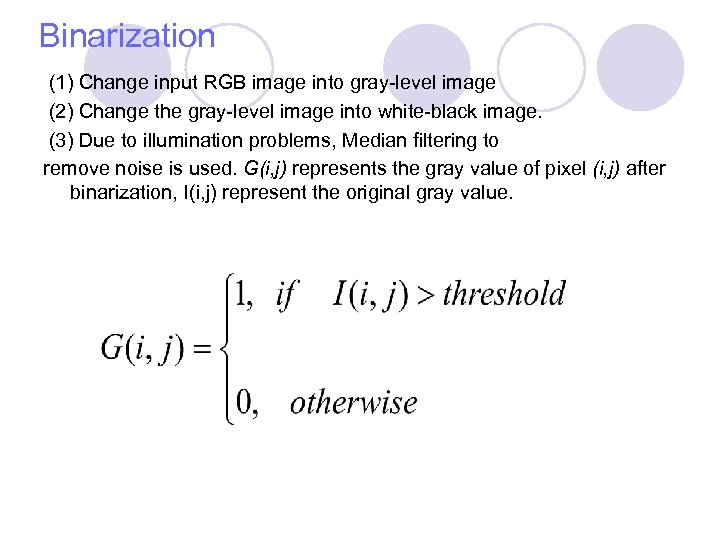Binarization (1) Change input RGB image into gray-level image (2) Change the gray-level image into white-black image. (3) Due to illumination problems, Median filtering to remove noise is used. G(i, j) represents the gray value of pixel (i, j) after binarization, I(i, j) represent the original gray value.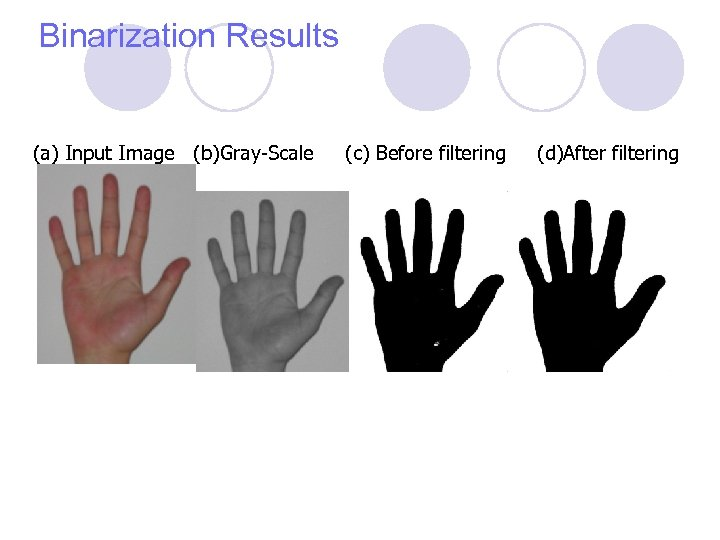Binarization Results (a) Input Image (b)Gray-Scale (c) Before filtering (d)After filtering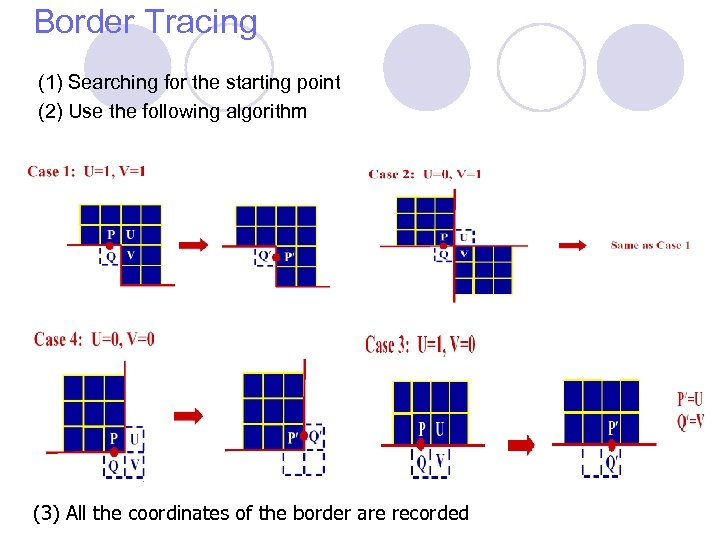Border Tracing (1) Searching for the starting point (2) Use the following algorithm (3) All the coordinates of the border are recorded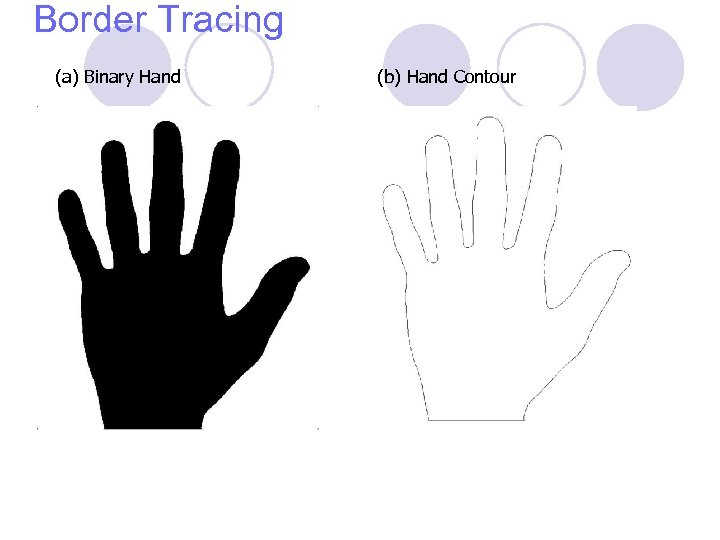Border Tracing (a) Binary Hand (b) Hand Contour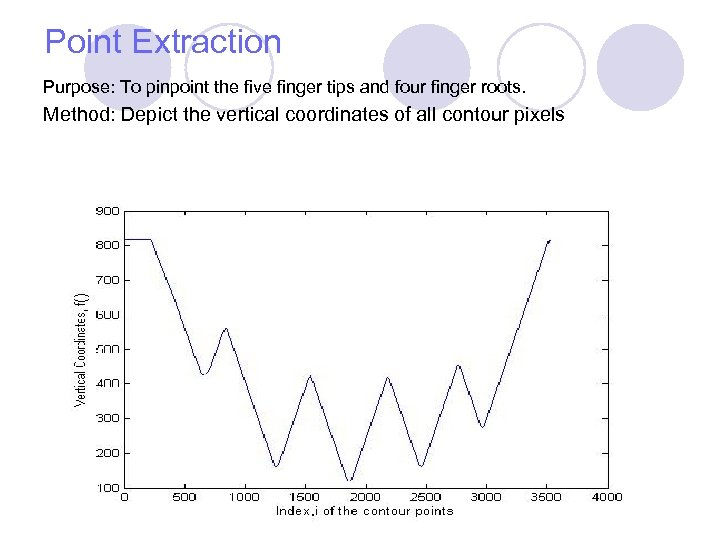Point Extraction Purpose: To pinpoint the five finger tips and four finger roots. Method: Depict the vertical coordinates of all contour pixels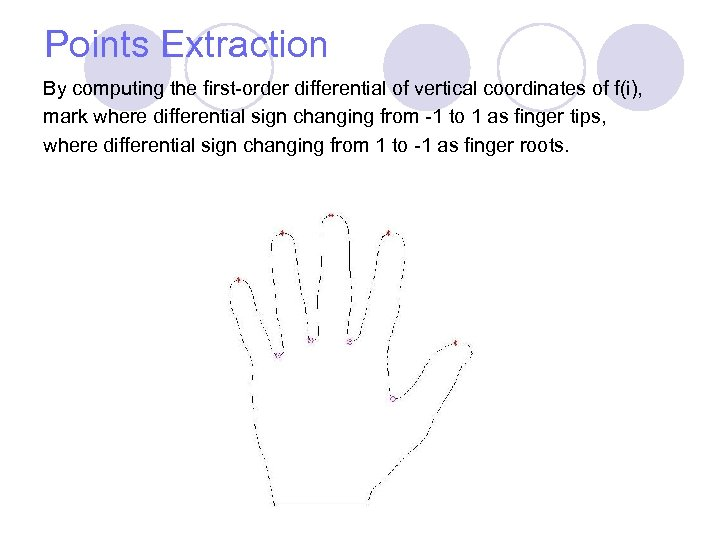Points Extraction By computing the first-order differential of vertical coordinates of f(i), mark where differential sign changing from -1 to 1 as finger tips, where differential sign changing from 1 to -1 as finger roots.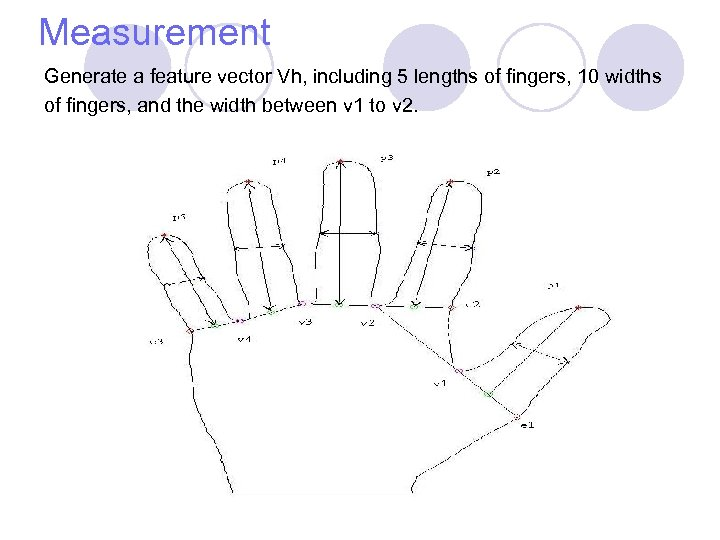Measurement Generate a feature vector Vh, including 5 lengths of fingers, 10 widths of fingers, and the width between v 1 to v 2.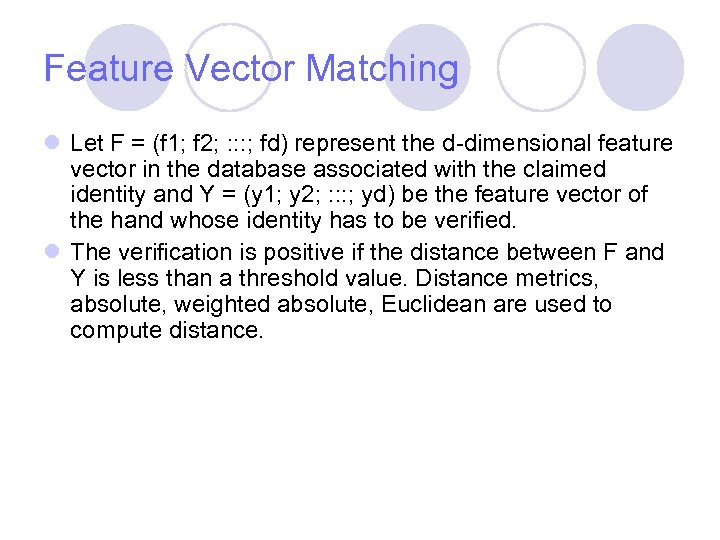Feature Vector Matching l Let F = (f 1; f 2; : : : ; fd) represent the d-dimensional feature vector in the database associated with the claimed identity and Y = (y 1; y 2; : : : ; yd) be the feature vector of the hand whose identity has to be verified. l The verification is positive if the distance between F and Y is less than a threshold value. Distance metrics, absolute, weighted absolute, Euclidean are used to compute distance.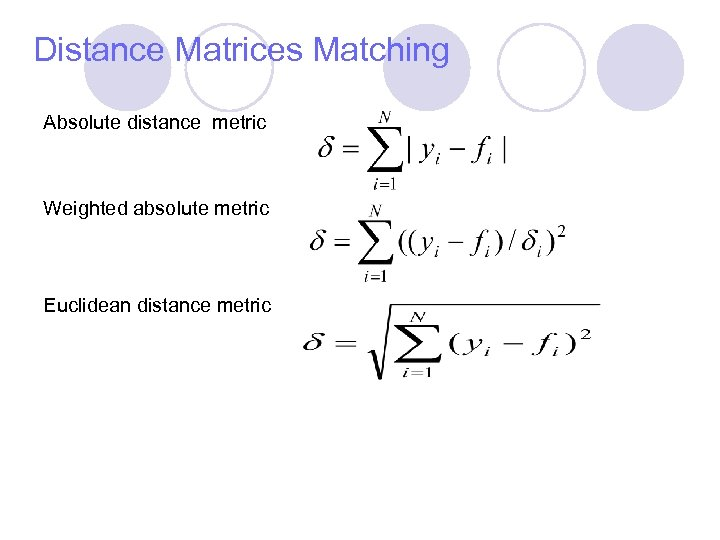Distance Matrices Matching Absolute distance metric Weighted absolute metric Euclidean distance metric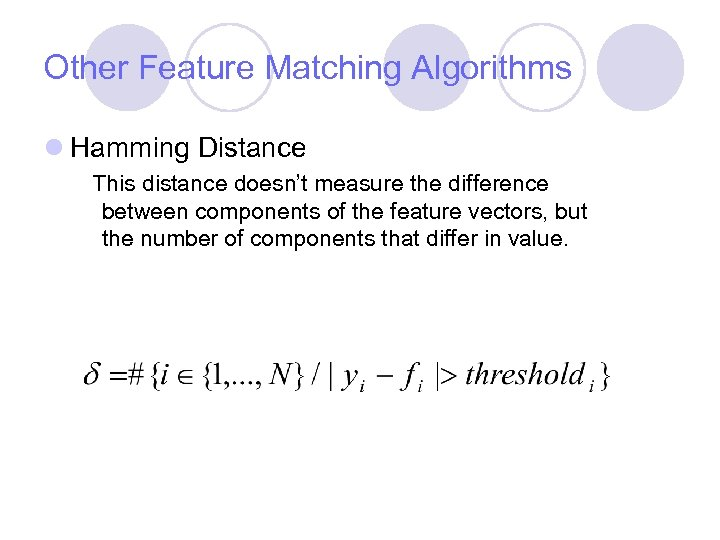Other Feature Matching Algorithms l Hamming Distance This distance doesn’t measure the difference between components of the feature vectors, but the number of components that differ in value.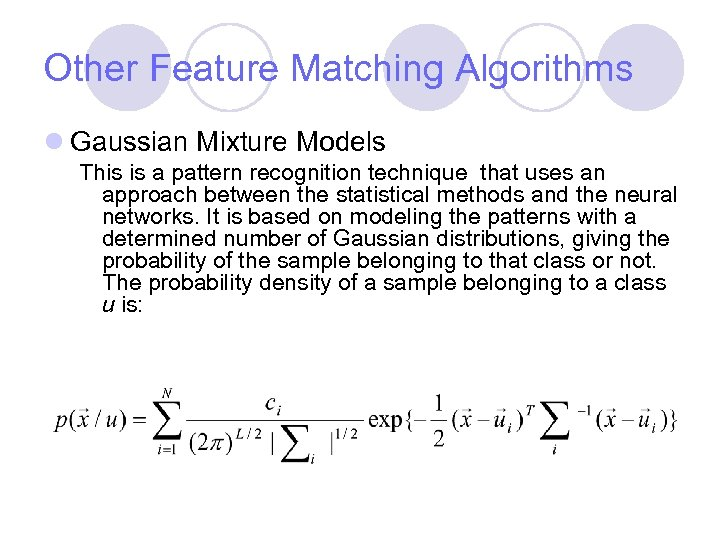Other Feature Matching Algorithms l Gaussian Mixture Models This is a pattern recognition technique that uses an approach between the statistical methods and the neural networks. It is based on modeling the patterns with a determined number of Gaussian distributions, giving the probability of the sample belonging to that class or not. The probability density of a sample belonging to a class u is: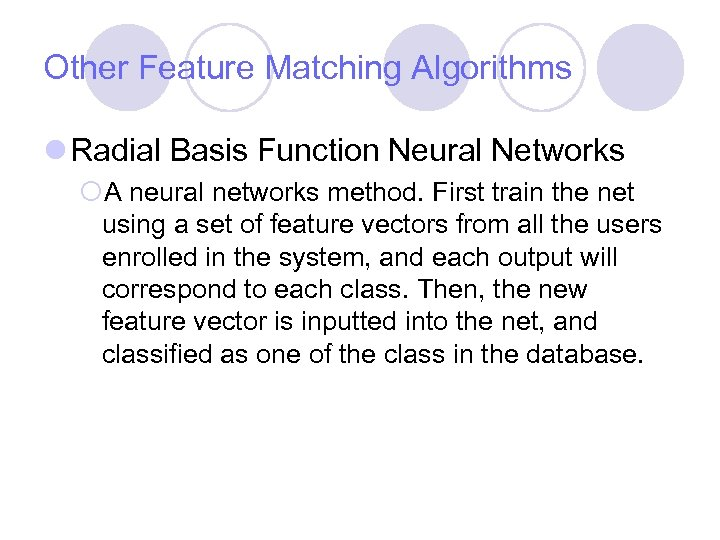Other Feature Matching Algorithms l Radial Basis Function Neural Networks ¡A neural networks method. First train the net using a set of feature vectors from all the users enrolled in the system, and each output will correspond to each class. Then, the new feature vector is inputted into the net, and classified as one of the class in the database.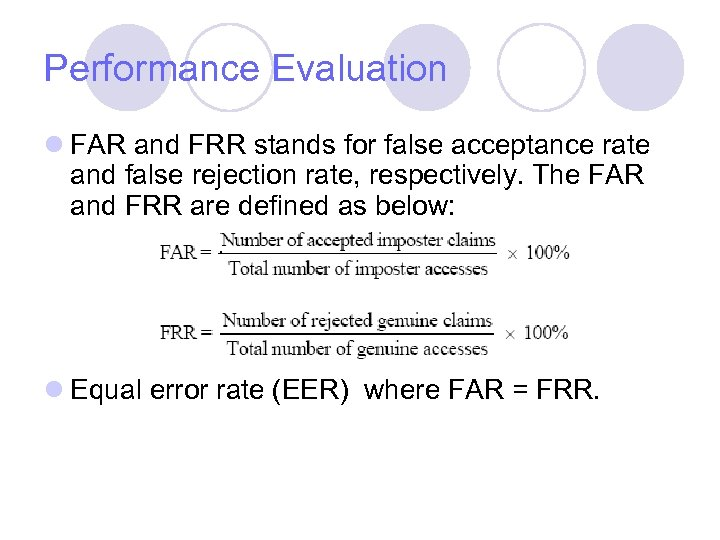Performance Evaluation l FAR and FRR stands for false acceptance rate and false rejection rate, respectively. The FAR and FRR are defined as below: l Equal error rate (EER) where FAR = FRR.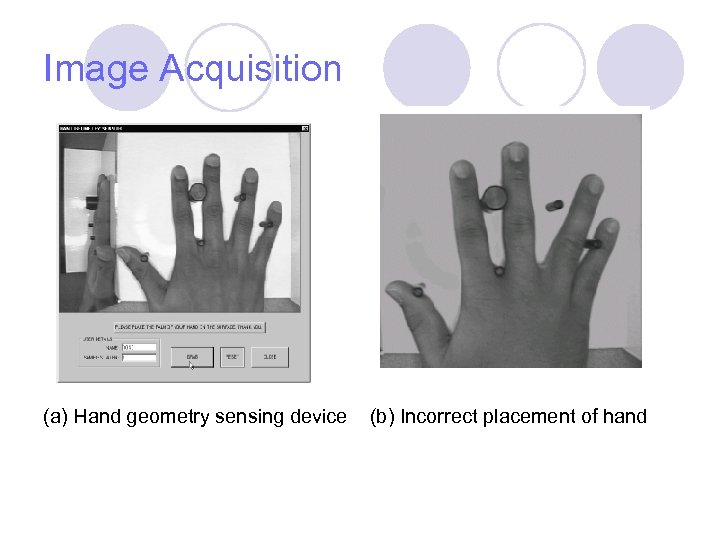Image Acquisition (a) Hand geometry sensing device (b) Incorrect placement of hand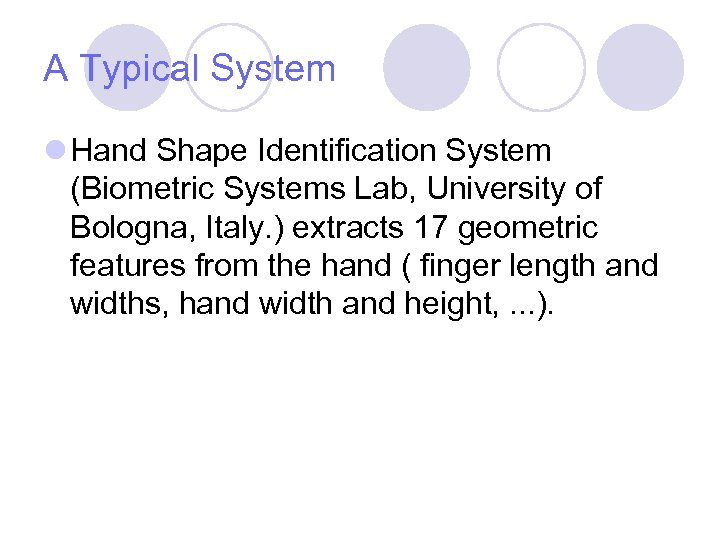A Typical System l Hand Shape Identification System (Biometric Systems Lab, University of Bologna, Italy. ) extracts 17 geometric features from the hand ( finger length and widths, hand width and height, . . . ).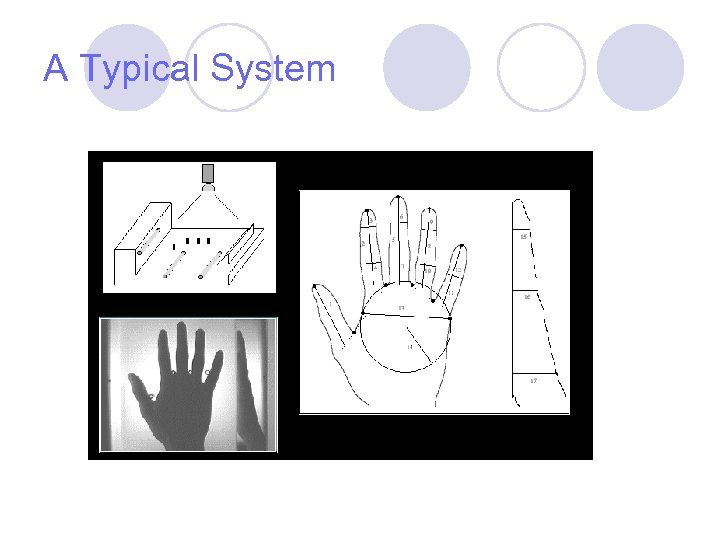A Typical System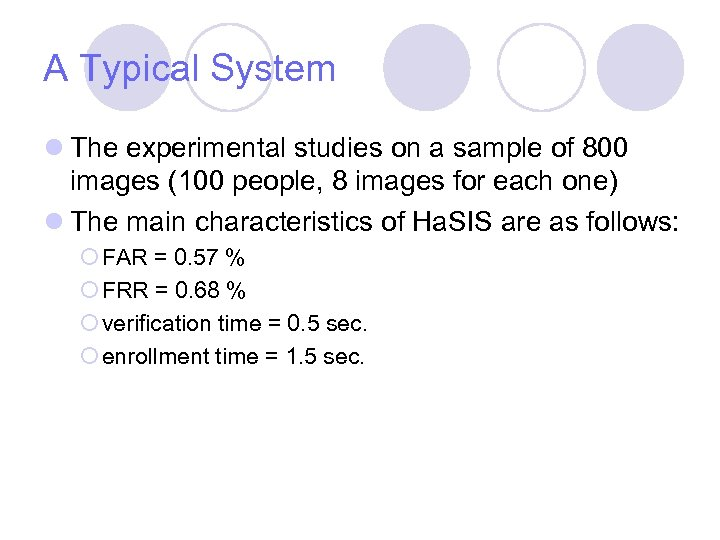A Typical System l The experimental studies on a sample of 800 images (100 people, 8 images for each one) l The main characteristics of Ha. SIS are as follows: ¡ FAR = 0. 57 % ¡ FRR = 0. 68 % ¡ verification time = 0. 5 sec. ¡ enrollment time = 1. 5 sec.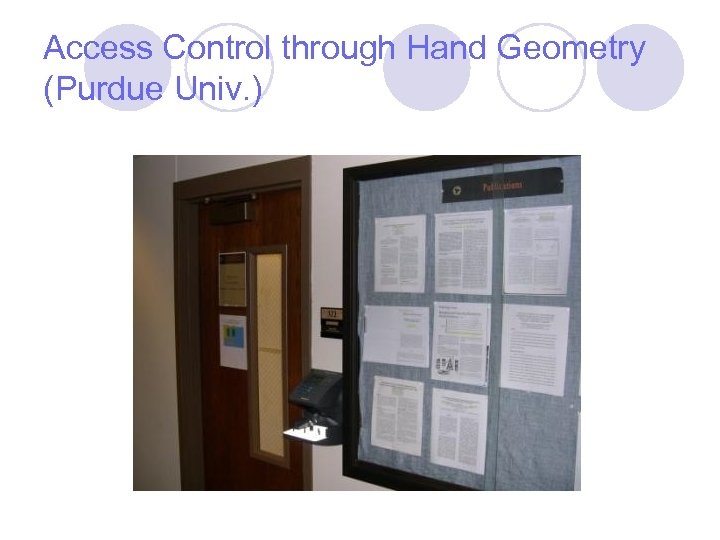Access Control through Hand Geometry (Purdue Univ. )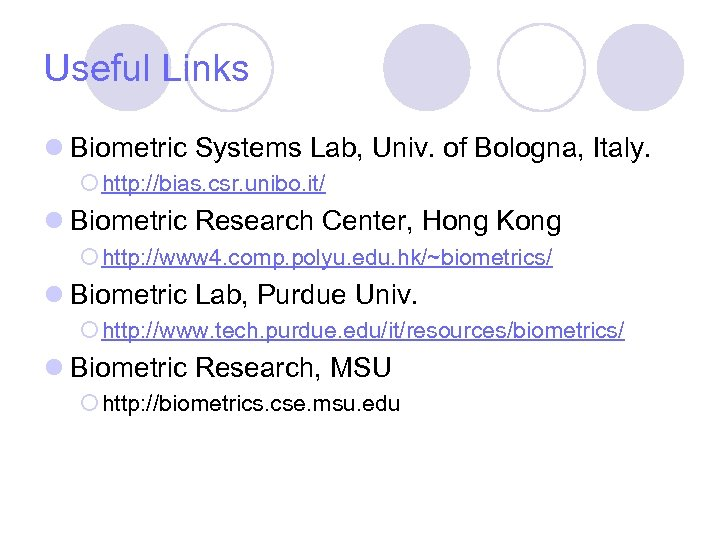Useful Links l Biometric Systems Lab, Univ. of Bologna, Italy. ¡ http: //bias. csr. unibo. it/ l Biometric Research Center, Hong Kong ¡ http: //www 4. comp. polyu. edu. hk/~biometrics/ l Biometric Lab, Purdue Univ. ¡ http: //www. tech. purdue. edu/it/resources/biometrics/ l Biometric Research, MSU ¡ http: //biometrics. cse. msu. edu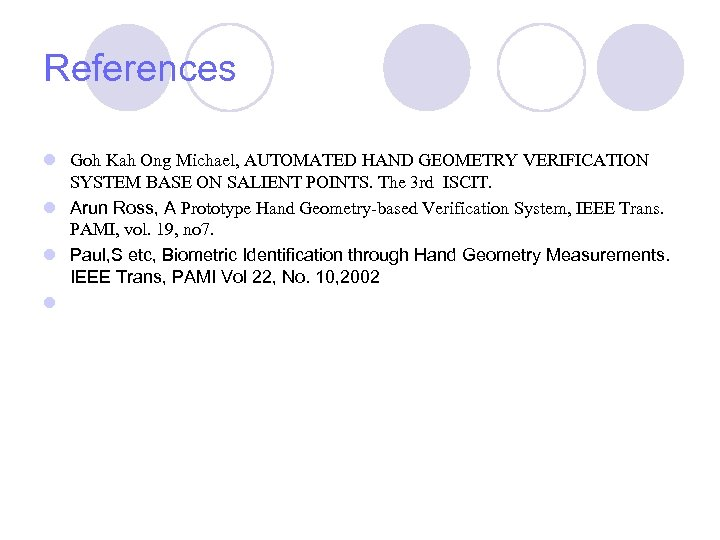References l Goh Kah Ong Michael, AUTOMATED HAND GEOMETRY VERIFICATION SYSTEM BASE ON SALIENT POINTS. The 3 rd ISCIT. l Arun Ross, A Prototype Hand Geometry-based Verification System, IEEE Trans. PAMI, vol. 19, no 7. l Paul, S etc, Biometric Identification through Hand Geometry Measurements. IEEE Trans, PAMI Vol 22, No. 10, 2002 l